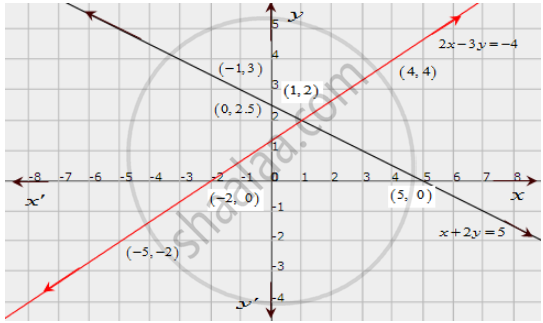Share

# Solve Graphically Each of the Following Systems of Linear Equations. Also, Find the Coordinates of the Points Where the Lines Meet the Axis Of X In Each System. X + 2y = 5 2x − 3y = −4 - CBSE Class 10 - Mathematics

ConceptGraphical Method of Solution of a Pair of Linear Equations

#### Question

Solve graphically each of the following systems of linear equations. Also, find the coordinates of the points where the lines meet the axis of x in each system.

x + 2y = 5
2x − 3y = −4

#### Solution

The given equations are

x + 2y = 5 .............(i)

2x − 3y = −4 ..............(ii)

The two points satisfying (i) can be listed in a table as,

 x 0 -1 y 2.5 3

The two points satisfying (ii) can be listed in a table as,

 x 4 -5 y 4 -2

Now, the graph of equations (i) and (ii) can be drawn as,It is seen that the solution of the given system of equations is given by x = 1, y = 2.

Also, it is observed that the coordinates of the points where the lines (i) and (ii) meet the x-axis are (5,0) and (-2,0) respectively.

Is there an error in this question or solution?

#### APPEARS IN

Solution Solve Graphically Each of the Following Systems of Linear Equations. Also, Find the Coordinates of the Points Where the Lines Meet the Axis Of X In Each System. X + 2y = 5 2x − 3y = −4 Concept: Graphical Method of Solution of a Pair of Linear Equations.
S